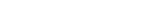MathExamle.com# Addition Subtraction Multiplication Division of decimals

Create worksheets of mathematical examples with addition, subtraction, multiplication and division of decimals and integers, select type of a generator and viewing some samples of task

Creates examples with operations of addition, subtraction, multiplication and division of decimals, the answers are always positive

3 Variants 4 Levels
 472.262-2.926÷4.18= 0.66÷0.825+891.392= 342.72÷21.76-3.758= 743.72+1.615×72=
Variant: 1 Level: Hard

Creates examples with operations of addition, subtraction, multiplication and division of decimals, the answers can be negative

3 Variants 4 Levels
 21.6÷24-93.04= 1.8×12-5.6= -2.6+7×0.8= -23.04÷72-89.74=
Variant: 1 Level: Normal

Creates expressions with the operation of rounding decimals

3 Variants 3 Levels
 44.72-3.35≈ 62.56÷3.03≈ 97.77×0.8≈ 9.41×9.25≈
Variant: A Level: Normal